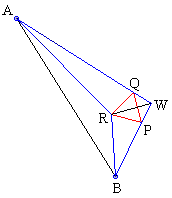## Morley's MiracleAnother proof

Several proofs of Morley's theorem start with a construction of an equilateral triangle with one vertex at the incenter of a given triangle and the other two vertices on its sides. I'll first prove that lemma and then demonstrate how it can be used in proving Morley's theorem.The importance of the lemma stems from an observation that, for example, in ΔABW, lines AR and BR are respective bisectors of angles A and B. Therefore, R is the incenter of that triangle and RW bisects ∠AWB. Similar assertions hold for triangles ACV and BCU.

### Lemma

RW also bisects ∠PRQ.

To prove that this is so, start with a "blank" triangle ABW. Locate its incenter R and draw two lines RP and RQ at angles 30° to the bisector RW. Then ΔPQR is equilateral.

Indeed, by construction, bisector RW also bisects ∠PRQ. Therefore, triangles QRW and PRW are equal, by ASA. It follows that ΔPQR is isosceles (PR = QR) with the apex ∠PRQ being 60° by construction, which implies that the triangle PQR is equilateral.

As the following proof of Morley's theorem shows, the triangle constructed in Lemma is in fact Morley's triangle. Therefore, Lemma gives us an extra information that helps in finding the angles of the triangles that appear in Morley's configuration. In particular, Figure 1 in Newman's proof and the implicit justification of angles used in Conway's proof, both depend on this lemma.Note that if the triangle PQR is obtained as described in the lemma, then ∠BRP = (A + π)/3. This confirms with (and explains the selection of) the angles on Figure 1 for Morley's triangle. The same is true for the angles BPR, AQR, and ARQ. A priori there is no assurance however that the point P obtained by this construction will coincide with the point P which is the intersection of two trisectors inside ΔBCU. The same, of course, applies to the point Q.

We may now consider three triangles ABW, BCU, and ACV. In each, according to the recipe spelled out in the lemma, construct an equilateral triangle. Generally speaking, we thus get three equilateral triangles. I want to show that they actually coalesce into one - Morley's triangle - which will prove the trisector theorem.

From the observation on the values of the involved angles, the sides of the three equilateral triangles are parallel. To distinguish between them I use letters b (black), g (green), and r (red) to denote both the triangles themselves and the lengths of their sides. In principle, we have to consider three cases of relative positions of the triangles b, g, r.The diagram shows that, in every case, we get some contradiction, e.g., b > r and simultaneously r > b in the first case. (If two triangles coincide then we should only consider the first two cases to show that the third triangle is bound to coincide with the other two.)### Morley's Miracle

#### Invalid proofs